Subsections

# 10.1BinaryHeap: An Implicit Binary Tree

Our first implementation of a (priority) Queue is based on a technique that is over four hundred years old. Eytzinger's method allows us to represent a complete binary tree as an array by laying out the nodes of the tree in breadth-first order (see Section 6.1.2). In this way, the root is stored at position 0, the root's left child is stored at position 1, the root's right child at position 2, the left child of the left child of the root is stored at position 3, and so on. See Figure 10.1.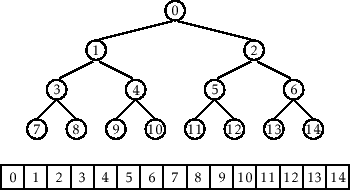If we apply Eytzinger's method to a sufficiently large tree, some patterns emerge. The left child of the node at index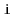is at index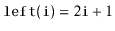and the right child of the node at indexis at index. The parent of the node at indexis at index.

    int left(int i) {
return 2*i + 1;
}
int right(int i) {
return 2*i + 2;
}
int parent(int i) {
return (i-1)/2;
}


A BinaryHeap uses this technique to implicitly represent a complete binary tree in which the elements are heap-ordered: The value stored at any indexis not smaller than the value stored at index, with the exception of the root value,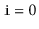. It follows that the smallest value in the priority Queue is therefore stored at position 0 (the root).

In a BinaryHeap, the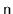elements are stored in an array:

    T[] a;
int n;


Implementing theoperation is fairly straightforward. As with all array-based structures, we first check to see if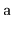is full (by checking if) and, if so, we grow. Next, we placeat locationand increment. At this point, all that remains is to ensure that we maintain the heap property. We do this by repeatedly swappingwith its parent until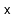is no longer smaller than its parent. See Figure 10.2.

    boolean add(T x) {
if (n + 1 > a.length) resize();
a[n++] = x;
bubbleUp(n-1);
return true;
}
void bubbleUp(int i) {
int p = parent(i);
while (i > 0 && compare(a[i], a[p]) < 0) {
swap(i,p);
i = p;
p = parent(i);
}
}Implementing theoperation, which removes the smallest value from the heap, is a little trickier. We know where the smallest value is (at the root), but we need to replace it after we remove it and ensure that we maintain the heap property.

The easiest way to do this is to replace the root with the value, delete that value, and decrement. Unfortunately, the new root element is now probably not the smallest element, so it needs to be moved downwards. We do this by repeatedly comparing this element to its two children. If it is the smallest of the three then we are done. Otherwise, we swap this element with the smallest of its two children and continue.

    T remove() {
T x = a;
a = a[--n];
trickleDown(0);
if (3*n < a.length) resize();
return x;
}
void trickleDown(int i) {
do {
int j = -1;
int r = right(i);
if (r < n && compare(a[r], a[i]) < 0) {
int l = left(i);
if (compare(a[l], a[r]) < 0) {
j = l;
} else {
j = r;
}
} else {
int l = left(i);
if (l < n && compare(a[l], a[i]) < 0) {
j = l;
}
}
if (j >= 0)    swap(i, j);
i = j;
} while (i >= 0);
}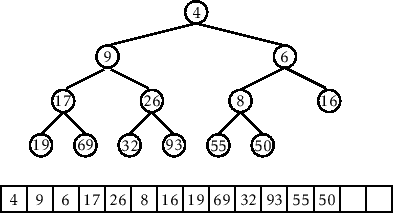As with other array-based structures, we will ignore the time spent in calls to, since these can be accounted for using the amortization argument from Lemma 2.1. The running times of bothandthen depend on the height of the (implicit) binary tree. Luckily, this is a complete binary tree; every level except the last has the maximum possible number of nodes. Therefore, if the height of this tree is, then it has at leastnodes. Stated another wayTaking logarithms on both sides of this equation givesTherefore, both the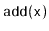and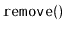operation run in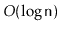time.

## 10.1.1 Summary

The following theorem summarizes the performance of a BinaryHeap:

Theorem 10..1   A BinaryHeap implements the (priority) Queue interface. Ignoring the cost of calls to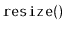, a BinaryHeap supports the operationsandintime per operation.

Furthermore, beginning with an empty BinaryHeap, any sequence ofandoperations results in a total oftime spent during all calls to.

opendatastructures.org